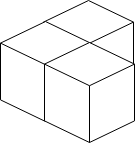615. Building Boxes

0

Hard

You have a cubic storeroom with dimensions n x n x n units. Your task is to arrange n boxes in this room, where each box is a cube with side length 1 unit. However, there are certain rules for placing the boxes: 1. The boxes can be placed anywhere on the floor of the room. 2. If box x is placed on top of box y, then each side of the four vertical sides of box y must either be adjacent to another box or to a wall. Given an integer n, you need to determine the minimum number of boxes that must touch the floor.Input Format

The first line of input contains an integer n.

Output Format

Output a single integer, which represents the minimum possible number of boxes touching the floor.

Example

Input

3

Output

3

Constraints

1 <= n <= 10^9